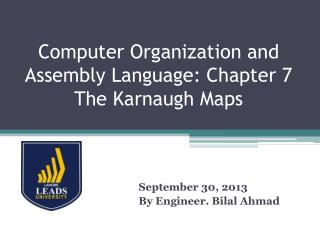DownloadDownload PresentationComputer Organization and Assembly Language: Chapter 7 The Karnaugh Maps

# Computer Organization and Assembly Language: Chapter 7 The Karnaugh Maps

Télécharger la présentation## Computer Organization and Assembly Language: Chapter 7 The Karnaugh Maps

- - - - - - - - - - - - - - - - - - - - - - - - - - - E N D - - - - - - - - - - - - - - - - - - - - - - - - - - -
##### Presentation Transcript

1. Computer Organization and Assembly Language: Chapter 7 The Karnaugh Maps September 30, 2013 By Engineer. Bilal Ahmad

2. Significance • K-Map is used to simplify Boolean Expressions. • The K-Map reduces the need of extensive calculation by taking the advantage of Humans Pattern Reorganization Capability. • The required Boolean Expressions are transferred on a truth table on a 2 dimensional grid where cells are ordered in gray code. • Do you know what is a gray code, write for 2 bits

3. Representation of K-Maps • Each cell represents one combination of input condition while each cell represents the output value. • K-Maps are used to simplify real world logic requirements so that they can be implemented by using a limited no of physical gates.

4. What do you know so far? • With the introduction of SOP and POS in chapter 6 you learned how to convert a truth table to Boolean Expression. • You have noticed the two layer simplification. • Yes it satisfied the condition and the architecture was simplified but can it is simplified further. • The answer to that is yes, you can use K-Map to simplify further but how? You will learn in this lecture.

5. An Example • Below is a truth table given and the resulted Boolean Expression.

6. Example Continued • The resulting Boolean Expression is;

7. Analysis of the Example • What you have learnt is that YES SOP and POS are used for the simplification to reduce the no. of logical gates but it is not the last stage of simplification. It can be further simplified and the no. of gates can be reduced further. • What this chapter will do is, it will present a graphical method to pair up products where this rule can be applied in order to drop out as many terms as possible.

8. K-Maps • It is the graphical representation of Truth Tables. • They consist of grid with one cell for each row of a truth table. The grid shown below is the two-by-two K-Map used to represent a truth table with two input variables.

9. How to Map a K-Map

10. Analysis • Have you noticed anything different while writing the combination in the previous slide? • The traditional way to count the binary values is 00,01,10 and 11. because we must use gray codes we have to avoid this pattern. Otherwise the end product will not be in the most simplified form.

11. Structure of K-Maps • K-Map is to rearrange truth table so that adjacent cells can be combined allowing for a term to drop out. • In other words the key to the effectiveness of the K-Map is that adjacent cell represents the output for a specific pattern of one’s and zero’s at the input and that to move to an adjacent cell.

12. Example and Simplification • Convert the 3 input truth table to its corresponding K-Map

13. Solution

14. Using K-Maps • We can derive an expression by using the K-Map similar to what we were doing for the SOP expressions. • The expression will be;

15. Using K-Maps Continued

16. Another Example • K-Map can go further for example;

17. How to effectively use K-Maps • So the key to effectively use K Maps is to find the largest group of adjacent cells containing ones. The larger the group the fewer products and inputs will be needed to create the Boolean Expression that produces the Truth Table. In the coming slides there will be some rules presented that should be obeyed.

18. How to Group in K-Maps

19. Grouping in K-Maps Continued

20. Example • Determine the Minimal Expression for the Truth Table;

21. Conversion to K-Map • From the Previous Truth Table Have you made a K-Map like this;

22. Few Announcements • Next week till Friday you have to submit your Reflective Log Books. • On the Next Page there is an assignment that has also to be submitted till next Friday. • Your midterm will come up to chapter 8. We have completed Chapter 7 today. • Good Luck, any thing after chapter 8 will not come in the mid term.

23. Assignment no 2